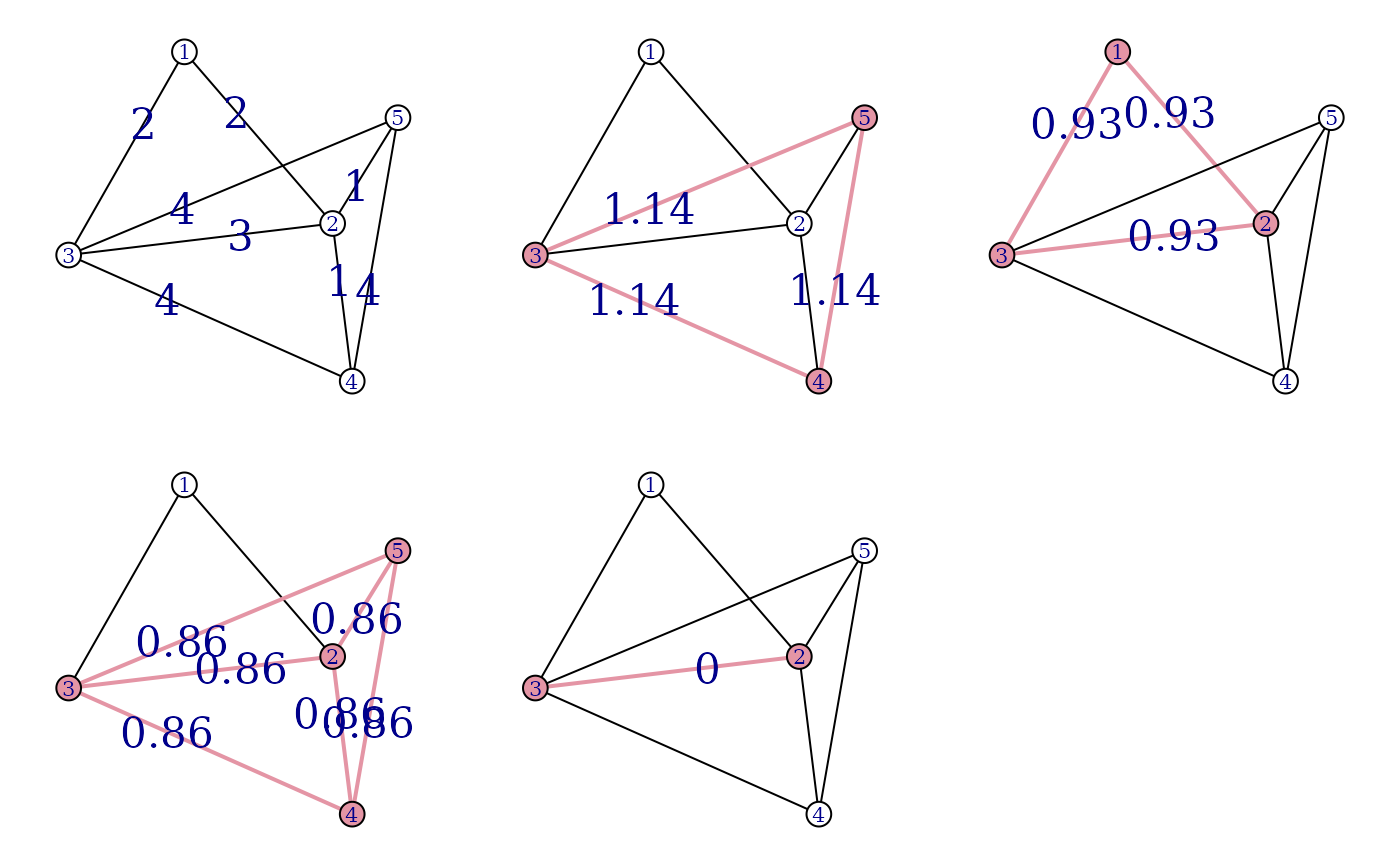Graphlet decomposition models a weighted undirected graph via the union of potentially overlapping dense social groups. This is done by a two-step algorithm. In the first step a candidate set of groups (a candidate basis) is created by finding cliques if the thresholded input graph. In the second step these the graph is projected on the candidate basis, resulting a weight coefficient for each clique in the candidate basis.

## Usage

graphlet_basis(graph, weights = NULL)

graphlet_proj(
graph,
weights = NULL,
cliques,
niter = 1000,
Mu = rep(1, length(cliques))
)

## Arguments

graph

The input graph, edge directions are ignored. Only simple graph (i.e. graphs without self-loops and multiple edges) are supported.

weights

Edge weights. If the graph has a weight edge attribute and this argument is NULL (the default), then the weight edge attribute is used.

cliques

A list of vertex ids, the graphlet basis to use for the projection.

niter

Integer scalar, the number of iterations to perform.

Mu

Starting weights for the projection.

## Value

graphlets() returns a list with two members:

cliques

A list of subgraphs, the candidate graphlet basis. Each subgraph is give by a vector of vertex ids.

Mu

The weights of the subgraphs in graphlet basis.

graphlet_basis() returns a list of two elements:

cliques

A list of subgraphs, the candidate graphlet basis. Each subgraph is give by a vector of vertex ids.

thresholds

The weight thresholds used for finding the subgraphs.

graphlet_proj() return a numeric vector, the weights of the graphlet basis subgraphs.

## Details

igraph contains three functions for performing the graph decomponsition of a graph. The first is graphlets(), which performed both steps on the method and returns a list of subgraphs, with their corresponding weights. The second and third functions correspond to the first and second steps of the algorithm, and they are useful if the user wishes to perform them individually: graphlet_basis() and graphlet_proj().

## Examples


## Create an example graph first
D1 <- matrix(0, 5, 5)
D2 <- matrix(0, 5, 5)
D3 <- matrix(0, 5, 5)
D1[1:3, 1:3] <- 2
D2[3:5, 3:5] <- 3
D3[2:5, 2:5] <- 1

g <- simplify(graph_from_adjacency_matrix(D1 + D2 + D3,
mode = "undirected", weighted = TRUE
))
V(g)$color <- "white" E(g)$label <- E(g)$weight E(g)$label.cex <- 2
E(g)$color <- "black" layout(matrix(1:6, nrow = 2, byrow = TRUE)) co <- layout_with_kk(g) par(mar = c(1, 1, 1, 1)) plot(g, layout = co) ## Calculate graphlets gl <- graphlets(g, niter = 1000) ## Plot graphlets for (i in 1:length(gl$cliques)) {
sel <- gl$cliques[[i]] V(g)$color <- "white"
V(g)[sel]$color <- "#E495A5" E(g)$width <- 1
E(g)[V(g)[sel] %--% V(g)[sel]]$width <- 2 E(g)$label <- ""
E(g)[width == 2]$label <- round(gl$Mu[i], 2)
E(g)$color <- "black" E(g)[width == 2]$color <- "#E495A5"
plot(g, layout = co)
}